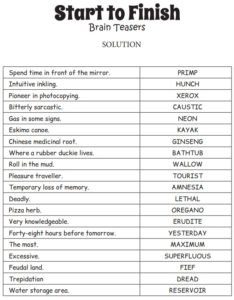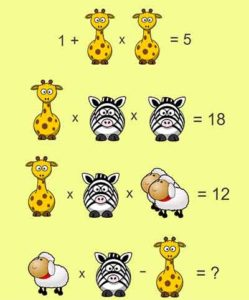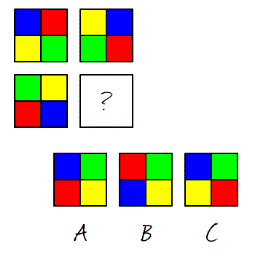Select Page

## Did you try each puzzle several times?

Here are the answers to Brain Practice, February, 2020

#### Start to Finish (Words puzzle)#### Girafe, Zebra and Sheep puzzle.Giraffe = 2
1 + 2 x 2 = 1 + 4 = 5

Zebra = 3
2 x 3 x 3 = 6 x 3 = 18

Sheep = 1 (two sheep = 2)
2 x 3 x (2 x 1) = 2 x 3 x 2 = 6 x 2 = 12

Result
1 x 3 – 2 = 3 – 2 = 1

#### Block PuzzleB is the correct solution

#### Did you Solve these?

You always follow me but I am rarely seen. What am I?
I am a queue

I am a word. I become longer when the third letter is removed. What am I?
I am a lounger

“One pace to the North. Two paces to the East. Two paces to the South. Two paces to the West. One pace to the North. What am I?”
I am a Square